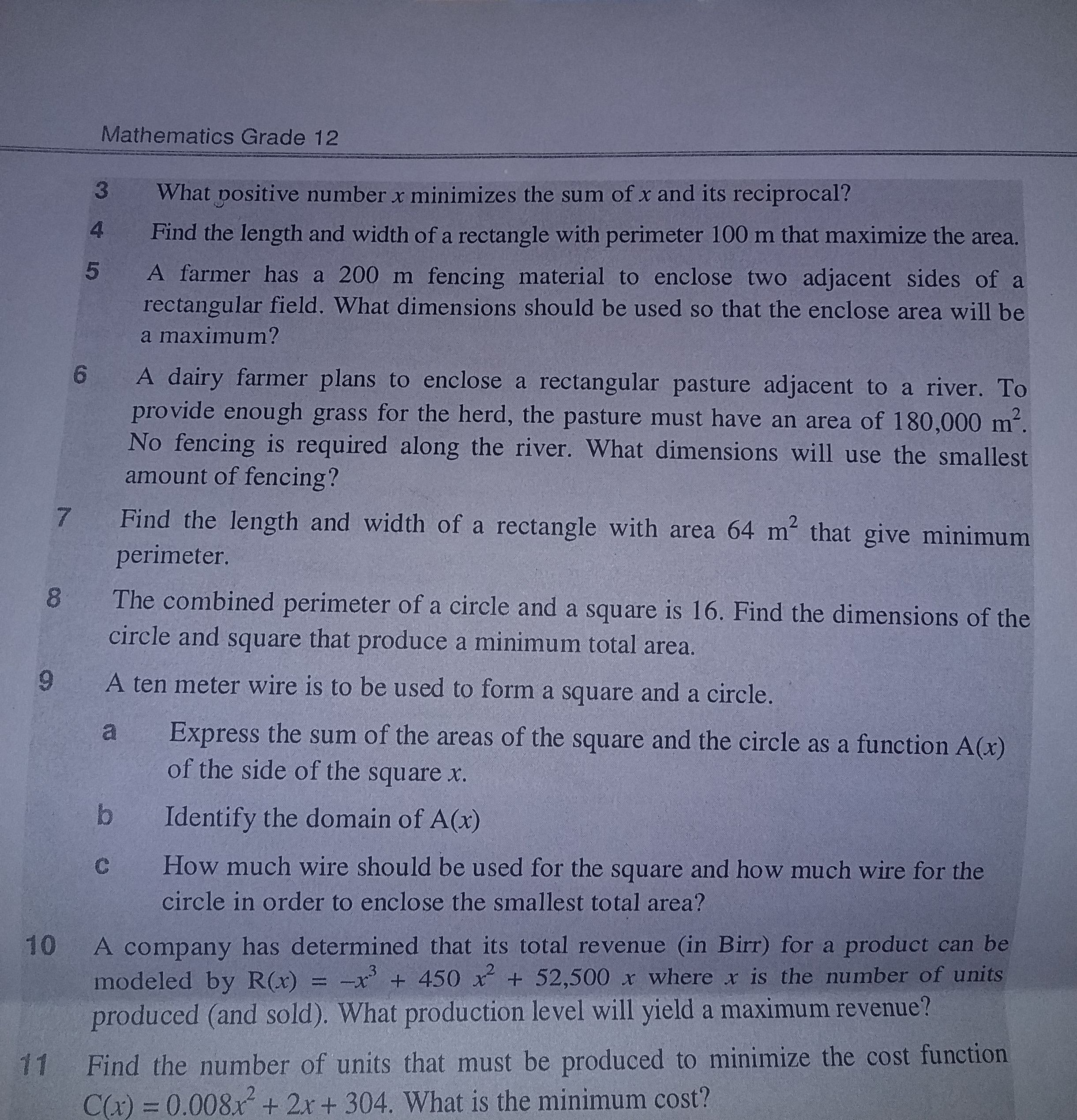nasriibraahim507

2022-04-07

A farmer has a 200 m fencingmaterial to enclose two adjacent sides of a rectangular field. What dimensions should be used so that the enclose area will be a maximum?nick1337

Expert

Let area be $A$ and the sides of rectangular field be $x$ and $y$;
So,

$A=x\cdot y$

Now, one side of the rectangle is already made with a fence.
There are $4$ sides, two sides of $x$ meters and two sides of $y$ meters. Let a side of $y$ meters be already fenced.

Then, the remaining three sides are to be fenced with a fence of  length,

So,

$2x+y=200$

$y=200-2x$
So,

$A=x\left(200-2x\right)$

$A=200x-2{x}^{2}$

For area to be maximum,

$\frac{dA}{dx}=0$ and $\frac{{d}^{2}A}{{dx}^{2}}<0$

Now,

$\frac{dA}{dx}=200-4x$

$200-4x=0$

$x=50$

Now,

$\frac{{d}^{2}A}{{dx}^{2}}=-4$

Which is negative

Thus, $x=50$ is a maximum.

Now,

$2x+y=200$

$2\left(50\right)+y=200$

$y=100$

Thus, the required area is:

Do you have a similar question?